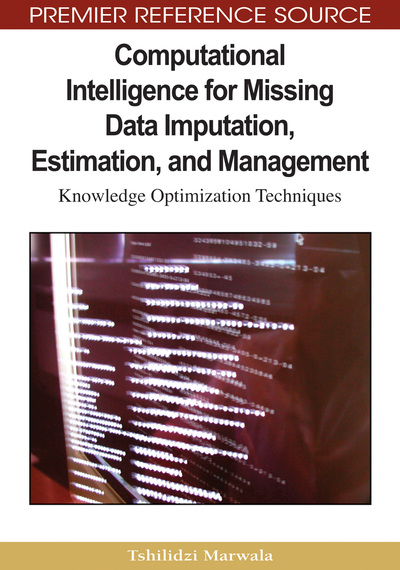# Emerging Missing Data Estimation Problems: Heteroskedasticity; Dynamic Programming and Impact of Missing Data

Tshilidzi Marwala
DOI: 10.4018/978-1-60566-336-4.ch013
OnDemand:
(Individual Chapters)
Available
\$37.50
No Current Special Offers

## Abstract

This chapter is divided into three parts: The first part presents a computational intelligence approach for predicting missing data in the presence of concept drift using an ensemble of multi-layered feed-forward neural networks. An algorithm that detects concept drift by measuring heteroskedasticity is proposed. Six instances prior to the occurrence of missing data are used to approximate the missing values. The algorithm is applied to simulated time series data sets resembling non-stationary data from a sensor. Results show that the prediction of missing data in non-stationary time series data is possible but is still a challenge. In the second part, an algorithm that uses dynamic programming and neural networks to solve the problem of missing data imputation is presented. A model that uses autoassociative neural networks and genetic algorithms is used as a basis; however, the neural networks are not trained using the entire data set. Data are broken up into granules and various models are created. The models are tested on a real dataset and the results show that the proposed method is effective in missing data estimation. In the third part of this chapter, a study of the impact of missing data estimation on fault classification in mechanical systems is undertaken. The fault classification task is implemented using the extension network as well as Gaussian mixture models. When the imputed values are used in the classification of faults using the extension networks, the fault classification accuracy of 95% is observed for single-missing-entry cases and 92% for two-missing-entry cases while the full database set is able to give classification accuracy of 97%. On the other hand, the Gaussian mixture model gives 94% for single-missing-entry cases and 92% for two-missing-entry cases while the full database set is able to give classification accuracy of 96%.
Chapter Preview
Top

## Introduction: Heteroskedasticity

The problem of missing data has intensively been researched but continues to be mainly unsettled. One of the causes for this is that the complexity of approximating missing variables is exceedingly reliant on the problem domain. This complexity, moreover, increases when data are missing in an on-line application where data have to be used as soon as they are obtained. A difficult characteristic of the missing data problem is when data are missing from a time series that exhibit non-stationarity. Most machine learning techniques and algorithms that have been developed thus far assume that data will continuously be obtainable. In addition, they assume that data conform to a stationary distribution.

Non-stationarity of a data essentially means that the character or the nature of the data is actually changing as a function of time. There are lots of non-stationary quantities in the natural world that fluctuate with time. Familiar examples include the stock market, weather, heartbeats, seismic waves as well as animal populations. There are some engineering and measurement systems that have been developed to detect and to quantify non-stationary quantities. Such instruments are not resistant to failures. These instruments include the wavelet methods which are time-frequency analysis methods (Marwala, 2002; Bujurke et al., 2007) and fractals methods (Lunga & Marwala, 2006a; Sadana, 2003&2005; Reiter, 1994). In this chapter, a procedure known as heteroskedasticity (Nelwamondo & Marwala, 2007a) is used to analyze concept drift with the aim of ensuring that the deployed missing data estimation method remains relevant even in the presence of the concept drift.

Computational intelligence techniques have previously been employed for analyzing non-stationary data such as the stock-market, nevertheless, the volatility of the data render the problem too complex to easily analyze. The 2003 Nobel Prize Laureates in Economics, Granger (2003) and Engle (1982) made an exceptional contribution to non-linear data analysis. Granger showed that long-established statistical methods could be deceiving if applied to variables that wander over time without returning to some long-run resting position. Engle (1982) on the other hand contributed a pioneering innovation of an Autoregressive Conditional Heteroskedasticity (ARCH), a technique to analyze and understand unpredictable movements in financial market prices. This method is, moreover, applicable to risk assessment. Dufour et al. (2004) introduced simulation-based finite-sample tests for Heteroskedasticity and ARCH effects. Hafner and Herwartz (2001) proposed option pricing under linear autoregressive dynamics, heteroskedasticity, and conditional leptokurtosis whereas Khalaf, Saphores, and Bilodeau (2003) introduced simulation-based exact jump tests in models with conditional heteroskedasticity and Inkmann (2000) introduced mis-specified heteroskedasticity in the panel probit model and made a comparison between Gaussian mixture models (GMM) and simulated maximum likelihood. Other work on the heteroskedasticity include its use on analyzing the performance of bootstrap neural tests for conditional heteroskedasticity in ARCH models (Siani & Peretti, 2007), pooling of cross-sectional and time-series data in the presence of heteroskedasticity as well as analyzing auto-correlation- and heteroskedasticity-consistent t-values with trending data (Krämer & Michels, 1997).

Numerous techniques for solving missing data problems have been developed and discussed at length in the literature (Little & Rubin, 1987). However, limited attempt has been made to approximate missing data in strictly non-stationary processes, where concepts change with time. The challenge with missing data problems in this application is that the approximation process must be complete before the next sample is taken. Moreover, more than one technique may be required to approximate the missing data due to drifting of concepts. As a result, the computational time needed, the amount of computational memory required and the model complexity may grow indefinitely as new data continually arrive (Last, 2002).

## Complete Chapter List

Search this Book:
Reset